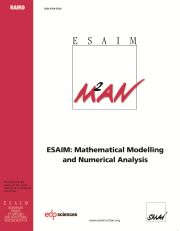Home
Hostname: page-component-684899dbb8-gbqfq Total loading time: 0.571 Render date: 2022-05-20T11:45:29.130Z Has data issue: true Feature Flags: { "shouldUseShareProductTool": true, "shouldUseHypothesis": true, "isUnsiloEnabled": true, "useRatesEcommerce": false, "useNewApi": true }ESAIM: Mathematical Modelling and Numerical Analysis

# On the convergence rate of approximation schemes forHamilton-Jacobi-Bellman Equations

Published online by Cambridge University Press:  15 April 2002

## Abstract

Using systematically a tricky idea of N.V. Krylov, we obtain general results on the rate of convergence of a certain class of monotone approximation schemes for stationary Hamilton-Jacobi-Bellman equations with variable coefficients. This result applies in particular to control schemes based on the dynamic programming principle and to finite difference schemes despite, here, we are not able to treat the most general case. General results have been obtained earlier by Krylov for finite difference schemes in the stationary case with constant coefficients and in the time-dependent case with variable coefficients by using control theory and probabilistic methods. In this paper we are able to handle variable coefficients by a purely analytical method. In our opinion this way is far simpler and, for the cases we can treat, it yields a better rate of convergence than Krylov obtains in the variable coefficients case.

Type
Research Article
Information

## Access options

Get access to the full version of this content by using one of the access options below. (Log in options will check for institutional or personal access. Content may require purchase if you do not have access.)

## References

Barles, G. and Souganidis, P.E., Convergence of approximation schemes for fully nonlinear second-order equations. Asymptotic Anal. 4 (1991) 271-283.
M. Bardi and I. Capuzzo-Dolcetta, Optimal Control and Viscosity Solutions of Hamilton-Jacobi-Bellman Equations. Birkhäuser, Boston (1997).
F. Bonnans and H. Zidani, Consistency of generalized finite difference schemes for the stochastic HJB equation. Preprint.
Camilli, F. and Falcone, M., An approximation scheme for the optimal control of diffusion processes. RAIRO Modél. Math. Anal. Numér. 29 (1995) 97-122. CrossRef
Capuzzo-Dolcetta, I., On a discrete approximation of the Hamilton-Jacobi equation of dynamic programming. Appl. Math. Optim. 10 (1983) 367-377. CrossRef
Crandall, M.G., Ishii, H. and Lions, P.-L., User's guide to viscosity solutions of second-order partial differential equations. Bull. Amer. Math. Soc. (N.S.) 27 (1992) 1-67. CrossRef
Crandall, M.G. and Lions, P.-L., Two approximations of solutions of Hamilton-Jacobi equations. Math. Comp. 43 (1984) 1-19. CrossRef
W.H. Fleming and H.M. Soner, Controlled Markov Processes and Viscosity Solutions. Springer-Verlag, New York (1993).
Ishii, H. and Lions, P.-L, Viscosity solutions of fully nonlinear second-order elliptic partial differential equations. J. Differential Equations 83 (1990) 26-78. CrossRef
E.R. Jakobsen and K.H. Karlsen, Continuous dependence estimates for viscosity solutions of fully nonlinear degenerate parabolic equations. To appear in J. Differential Equations.
Krylov, N.V., On the rate of convergence of finite-difference approximations for Bellman's equations. St. Petersbg Math. J. 9 (1997) 639-650.
Krylov, N.V., On the rate of convergence of finite-difference approximations for Bellman's equations with variable coefficients. Probab. Theory Relat. Fields 117 (2000) 1-16. CrossRef
H.J. Kushner, Numerical Methods for Approximations in Stochastic Control Problems in Continuous Time. Springer-Verlag, New York (1992).
Lions, P.-L., Existence results for first-order Hamilton-Jacobi equations. Ricerche Mat. 32 (1983) 3-23.
Lions, P.-L., Optimal control of diffusion processes and Hamilton-Jacobi-Bellman equations. Part I: The dynamic programming principle and applications. Comm. Partial Differential Equations 8 (1983) 1101-1174. CrossRef
Lions, P.-L., Optimal control of diffusion processes and Hamilton-Jacobi-Bellman equations. Part II: Viscosity solutions and uniqueness. Comm. Partial Differential Equations 8 (1983) 1229-1276. CrossRef
P.-L. Lions, Optimal control of diffusion processes and Hamilton-Jacobi-Bellman equations, Part III, in Nonlinear Partial Differential Equations and Appl., Séminaire du Collège de France, Vol. V, Pitman, Ed., Boston, London (1985).
Lions, P.-L. and Mercier, B., Approximation numérique des équations de Hamilton-Jacobi-Bellman. RAIRO Anal. Numér. 14 (1980) 369-393.
Menaldi, J.L., Some estimates for finite difference approximations. SIAM J. Control Optim. 27 (1989) 579-607. CrossRef
Souganidis, P.E., Approximation schemes for viscosity solutions of Hamilton-Jacobi equations. J. Differential Equations 59 (1985) 1-43. CrossRef

# Save article to Kindle

Note you can select to save to either the @free.kindle.com or @kindle.com variations. ‘@free.kindle.com’ emails are free but can only be saved to your device when it is connected to wi-fi. ‘@kindle.com’ emails can be delivered even when you are not connected to wi-fi, but note that service fees apply.

Find out more about the Kindle Personal Document Service.

On the convergence rate of approximation schemes for Hamilton-Jacobi-Bellman Equations
Available formats
×

# Save article to Dropbox

To save this article to your Dropbox account, please select one or more formats and confirm that you agree to abide by our usage policies. If this is the first time you used this feature, you will be asked to authorise Cambridge Core to connect with your Dropbox account. Find out more about saving content to Dropbox.

On the convergence rate of approximation schemes for Hamilton-Jacobi-Bellman Equations
Available formats
×

# Save article to Google Drive

To save this article to your Google Drive account, please select one or more formats and confirm that you agree to abide by our usage policies. If this is the first time you used this feature, you will be asked to authorise Cambridge Core to connect with your Google Drive account. Find out more about saving content to Google Drive.

On the convergence rate of approximation schemes for Hamilton-Jacobi-Bellman Equations
Available formats
×
×